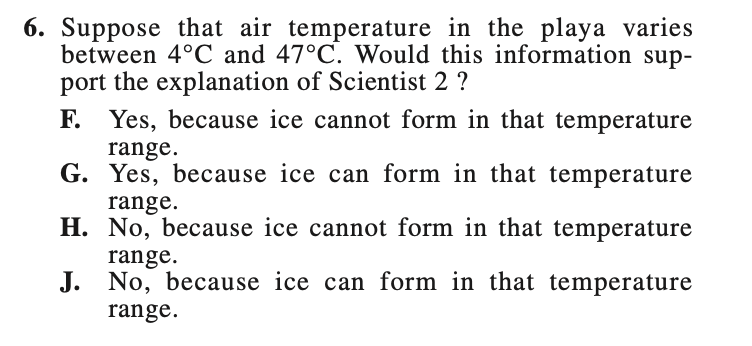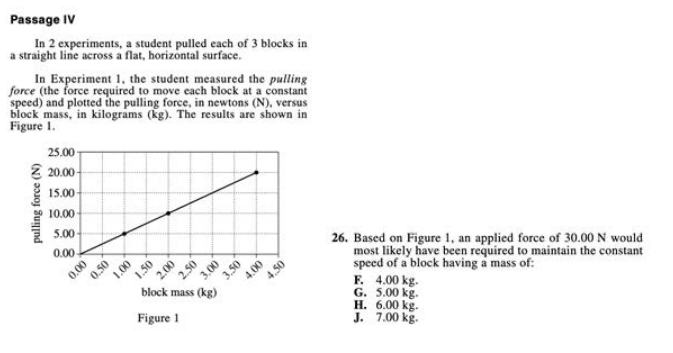Angelica Sirotin
4

# 5 ACT Science Formulas You Need to Know

### Do you know how to improve your profile for college applications?

See how your profile ranks among thousands of other students using CollegeVine. Calculate your chances at your dream schools and learn what areas you need to improve right now — it only takes 3 minutes and it's 100% free.

## What’s Covered

The ACT science section can be intimidating for many students.  Unless you’re a science whiz, the charts and graphs look like hieroglyphics. But there are certain scientific concepts that you can learn about in advance to help ease your mind when you get to test day. CollegeVine has identified the most important formulas every student should know.

The ACT Science section consists of six to seven paragraphs and 40 questions, all of which must be completed within 35 minutes. The test addresses topics such as biology, chemistry, physics, and the Earth/space sciences. This section of the ACT can be broken down into four subject areas: data representation, investigative procedures, patterns and relationships among scientific phenomena, and inquiry and reasoning skills.

You’ll need to use both inductive and deductive reasoning to answer the questions correctly. Sometimes you will be asked why something happens in a particular circumstance, other times you’ll need to determine how a result was obtained.

As with all standardized tests, proper preparation can greatly increase your test-taking ability and consequently your scores!

Here are the top six ACT science formulas every student needs to know. You should understand their meaning and be able to calculate values when needed.

## ACT Science Formulas You Need to Know

### 1.  Freezing and Boiling Point

Questions about temperature are common on the ACT science section. Many students will need to know the temperature of a substance at a certain point during an experiment, or how a change in temperature can affect a reaction. There are two formulas you should know: one for determining the freezing point of water and one for finding out its boiling point.

Freezing Point = 32° F or 0° C

Boiling Point = 212° F or 100°C

Let’s take a look at an example ACT question:The correct answer is F. If the air temperature of the playa ranges from 4℃ to 47℃, then, then the water will remain liquid because the freezing point of water is 0℃.  We know that Scientist 2 states that the “temperature in the playa does not get cold enough for ice to form”, which would uphold this statement by scientist 2.

### 2. Density

Density is defined as the mass per unit volume of a substance. In other words, an object will sink more slowly in a fluid with greater density. The relationship between mass and volume on the ACT is as follows:

Formula for Density:

Density = mass/volume

This question specifically states “If the temperatures…are raised…and if the object neither expands nor contracts with the change in temperature, will the object more likely sink or remain afloat?” You can use the density formula and your understanding of density to work through the question below:D is the correct response. The object will sink because the water will become less dense than the object if the object does not expand. Increasing the temperature will result in expanding the object.

### 3. Calculations With Moles

Moles are defined as the amount of substance containing Avogadro’s number of particles, given as 6.022 x 10^23. Mole calculations are common on the ACT science section.

Let’s take a look at an example ACT question:The correct answer is J. We know that 1 mmol of CO2 has a mass of 0.044 grams. Therefore, any trial that yielded a minimum of 0.044 grams of CO2 produced 1 mmol of CO2. Since all 8 of the trials yielded over 0.044 grams of CO2, J can be the only correct answer.

## Discover your chances at hundreds of schools

Our free chancing engine takes into account your history, background, test scores, and extracurricular activities to show you your real chances of admission—and how to improve them.

### 4. Slope Formula

This formula is used to determine the slope of a line. The x and y values of each data point allow you to find the corresponding m and b (m = “y” -intercept; b = “x” -intercept). Then, it’s as simple as plugging those numbers into this formula:

Slope Formula:

m=y2-y1/x2-x1

Let’s take a look at an example ACT question:The correct answer is B. In order to answer this question, you need to know that acceleration is the rate of change of speed. Finding the slope of the line in Figure 2 will provide us with the rate of change of speed, which is also known as acceleration. To find the slope, we can pick two points of our liking. If we pick (0,0) – meaning at time 0, the speed is 0 m/s – and pick (3,15) – meaning at 3 seconds, the speed is 15 m/s – we can use this information to deduce the following:

m = 15 – 0 / 3 – 0 = 5 m / sec^2 so B is the correct answer.

### 5. Force Formula

Your experiences with Newton’s Laws of Motion should enable you to answer this question correctly. Just remember that acceleration (the rate of change of velocity) is the same as force, which means that acceleration is directly proportional to net force.

F = m * a

This formula states that “force” equals mass times acceleration. The ACT expects you to know this equation, so you can answer ACT science questions correctly. Let’s take a look at an example ACT question:The correct answer is H. We can use the information obtained from the previous question on Slope to answer this question. Because we know acceleration is equal to 5, we know F = 5M. Therefore, we can plug in 30 for F, yielding 30 = 5M. This produces 6.00 kg as a result, meaning that H can be the only correct answer.

Selective colleges use a metric called the Academic Index (AI) to represent the strength of applicants’ grades and test scores. If your AI is too low, a school may not even review the rest of your application.

We’ve made it easy to understand the impact of your ACT score by creating a free Admissions Chances Calculator. This calculator will let you know how your score stacks up against other applicants’, and give you tips on improving the rest of your profile, including grades and extracurriculars.

You can also search for schools based on preferences like location, major, cost, and more. Give it a try to get a jumpstart on your college strategy.

## More ACT Science Resources

15 Hard ACT Science Questions to Improve Your Score

A Guide to the Science Section of the ACT

10 Tips for Acing the ACT Science Section

Guide to the Conflicting Viewpoints Passage on the ACT Science TestShort Bio
Angelica is 2020 grad of the University of Minnesota with a degree in quantitative economics. She is a Founder and CEO of a strategic business consulting firm that helps startups, small enterprise and entrepreneurs with their goals. In her free time, she likes to read and travel.

## Don't miss out on the best high school & college admissions resources!

Join thousands of students and parents getting exclusive high school, test prep, and college admissions information.